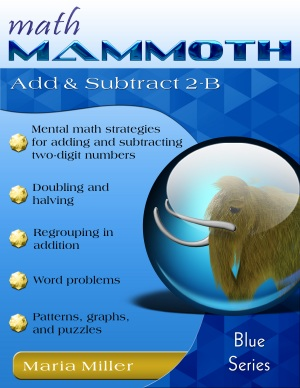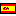^
You are here: HomeBlue Series → Add & Subtract 2-B

# Math Mammoth Add & Subtract 2-B95 pages

## Prices & ordering

Printed copy \$9.75

Printed copy £5.94
US shipping availableEste libro en español

Math Mammoth Add & Subtract 2-B is a continuation to the book Math Mammoth Add & Subtract 2-A. The goal of this book is to study addition and subtraction within 0-100, both mentally and in columns, especially concentrating on regrouping in addition (carrying) and in subtraction (borrowing).

### Mental math

Mental math is important because it builds number sense. This book includes many lessons that practice mental math. For example, the child practices adding and subtracting two-digit numbers when one of the numbers is a whole ten (problems such as 30 + 14, or 66 − 20).

Also studied are problems such as 36 + 8 or 45 + 9. These problems use the idea of going over ten as in problems 6 + 8 and 5 + 9. The child knows that 6 + 8 fills the first ten and is four more than the ten. He/she will learn to use that fact when adding 36 + 8. The sum 36 + 8 fills the next whole ten (40), and is four more than that, or 44.

### Regrouping in tens

Simultaneously with this, the child learns adding two-digit numbers in columns, and regrouping with tens, or "carrying," which is illustrated and explained in detail with the help of visual models. These visual models take the place of base-ten blocks or other manipulatives. You are welcome to use manipulatives to get the idea across, if you prefer. The main concept to understand is that 10 ones are regrouped to form a new ten, and this new ten is written using a little "1" in the tens column.

### Regrouping in subtraction

The next lessons teach subtracting in columns. First we only deal with the easy problems where you don't need to regroup (borrow). Then the following lessons practice in detail the process of regrouping or borrowing. You can use either term with your child, or even choose not to use either if you feel it is confusing. You can alternatively use the phrase "breaking a ten into ten ones."

First, the lesson Regrouping practices breaking down a ten into ten ones because we cannot subtract from the ones. It is crucial that the child understands what happens here. Otherwise, he/she might end up only memorizing the procedure, and will probably at some point misremember how it was done. That is why this lesson deals with the regrouping process in detail with plenty of visual exercises.

If you notice that the child does not understand the concept of regrouping, he/she may need more practice with concrete manipulatives or visual exercises before proceeding.

### More mental math

After learning regrouping, we practice mental subtraction in three separate lessons. One of them expounds on several methods for mental subtracting. Another is about Euclid's game—a fun game that also practices subtraction of two-digit numbers.

The PDF version of this book can be filled in on a computer, phone, or tablet, using the annotation tools found in many PDF apps. See more.

The video below shows a similar idea as to what is in the book about regrouping (borrowing).

## Feedback

Thank you SO much for the free download of Data & Graphs!! I had purchased several blue series books for my son but hadn't gotten that yet and it was exactly what I needed next.

I've been so impressed with your curriculum. My son is autistic and non-speaking and your curriculum has been accessible to him. He does not do well with manipulatives like some other curricula use because he seems to think in numbers like I do. My son is 6 and using Place Value 1&2, Add/Subtract 2A and 2B right now.

Lynn
June 2018

You might also be interested in:
• Math Mammoth Add & Subtract 2-A—a worktext about "easy" additions and subtractions within 0-100, memorizing the basic addition facts of single-digit numbers, and word problems (grades 1-2).
• Math Mammoth Add & Subtract 3—a worktext about adding and subtracting 3-digit numbers with regrouping and with mental math, estimation, and word problems (grades 2-3).
• Math Mammoth Place Value 2—a worktext about three-digit numbers (place value), comparing, ordering, rounding, bar graphs, and pictographs (grade 2).
• Math Mammoth Place Value 3—a worktext about four-digit place value, adding & subtracting 4-digit numbers mentally, rounding, estimating, and order of operations (grade 3).

## Better Yet - Bundle Deals!

*BONUS*: Buy any bundle listed above, and get the fully upgraded Soft-Pak software for FREE (includes 4 math and 2 language arts programs, complete with on screen and printable options).

## How and where to order

You can buy Math Mammoth books at:

• Here at MathMammoth.com website — simply use the "Add to cart" buttons you see on the product pages.
• Rainbow Resource carries printed copies for the Light Blue series books, plus several CDs (Light Blue and Blue series).
• At Teachers Pay Teachers you can purchase the Light Blue Series downloads, plus topical units.
• Lulu sells printed copies for most of the Math Mammoth materials (various series).

By purchasing any of the books, permission IS granted for the teacher (or parent) to reproduce this material to be used with his/her students in a teaching situation; not for commercial resale. However, you are not permitted to share the material with another teacher.

In other words, you are permitted to make copies for the students/children you are teaching, but not for other teachers' usage.

Math Mammoth books are PDF files. I recommend you use Adobe Reader to view them, including if you use a Mac. You can try other PDF viewers, but they may at times either omit or mess up some of the images.
WAIT!

Receive my monthly collection of math tips & resources directly in your inbox — and get a FREE Math Mammoth book!You can unsubscribe at any time.

### Math Mammoth Tour

Confused about the different options? Take a virtual email tour around Math Mammoth! You'll receive:

An initial email to download your GIFT of over 400 free worksheets and sample pages from my books. Six other "TOURSTOP" emails that explain the important things and commonly asked questions concerning Math Mammoth curriculum. (Find out the differences between all these different-colored series!)

This way, you'll have time to digest the information over one or two weeks, plus an opportunity to ask me personally about the curriculum.
A monthly collection of math teaching tips & Math Mammoth updates (unsubscribe any time)### "Mini" Math Teaching Course

This is a little "virtual" 2-week course, where you will receive emails on important topics on teaching math, including:

- How to help a student who is behind
- Troubles with word problems
- Teaching multiplication tables
- Why fractions are so difficult
- The value of mistakes
- Should you use timed tests
- And more!

A GIFT of over 400 free worksheets and sample pages from my books right in the very beginning.A monthly collection of math teaching tips & Math Mammoth updates (unsubscribe any time)
Enter your email to receive math teaching tips, resources, Math Mammoth news & sales, humor, and more! I tend to send out these tips about once monthly, near the beginning of the month, but occasionally you may hear from me twice per month (and sometimes less often).• A GIFT of over 400 free worksheets and sample pages from my books.